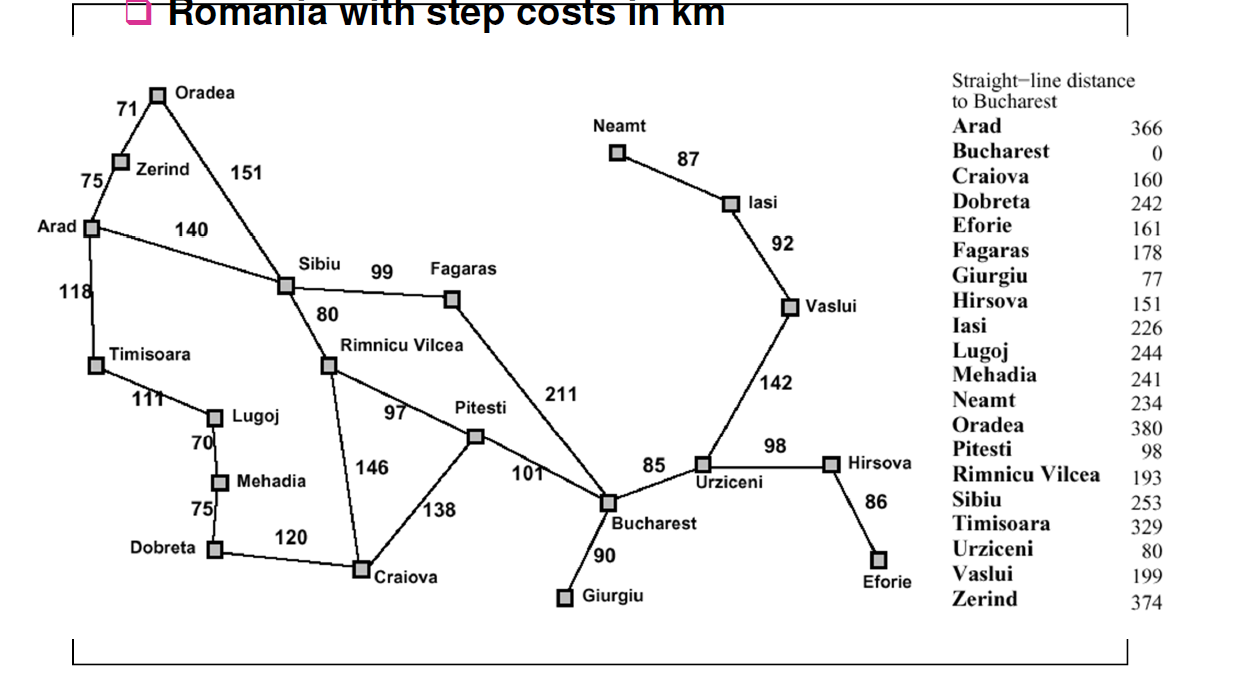5 views

In my textbook I noticed that both these algorithms work almost exactly the same, I am trying to understand what's the major difference between them.The textbook traversed this example using A* the same way it did with the best-first search.

Any help would be appreciated.

by (108k points)
edited by

 Best first search This algorithm visits the next state based on heuristics function f(n) = h with the lowest heuristic value (often called greedy). It doesn't consider the cost of the path to that particular state. All it cares about is that which next state from the current state has the lowest heuristics. A* search This algorithm visits the next state based on heuristics f(n) = h + g where h component is the same heuristics applied as in Best-first search but g component is the path from the initial state to the particular state. It doesn't choose the next state only with the lowest heuristics value but it selects the one that gives the lowest value when considering its heuristics and cost of getting to that state.

In your question, when you start from Arad you can go either straight to Sibiu (253km) or to the Zerind(374km) or Timisoara(329km). In this case, both algorithms choose Sibiu as it has a lower value f(n) = 253.

Now you can expand to either state back to Arad(366km) or Oradea(380km) or Fargas(178km) or Rimnicu Valcea(193km).

For best first search Faragas will have lowest f(n) = 178 but A* will have Rimnicu Vilcea f(n) = 220 + 193 = 413 where 220 is cost of getting to Rimnicu from Arad (140+80) and 193 is from Rimnicu to Bucharest but for Faragas it will be more as f(n) = 239 + 178 = 417.

So now clearly you can see best-first is greedy algorithm because it would choose a state with lower heuristics but higher overall cost as it doesn't consider the cost of getting to that state from the initial state.

If you want know about Artificial Intelligence and Deep Learning then you can watch this video:

Check more in-depth about Artificial Intelligence from this AI Course.